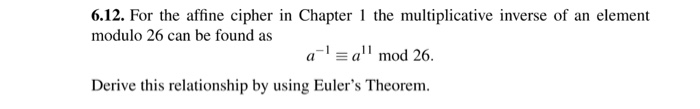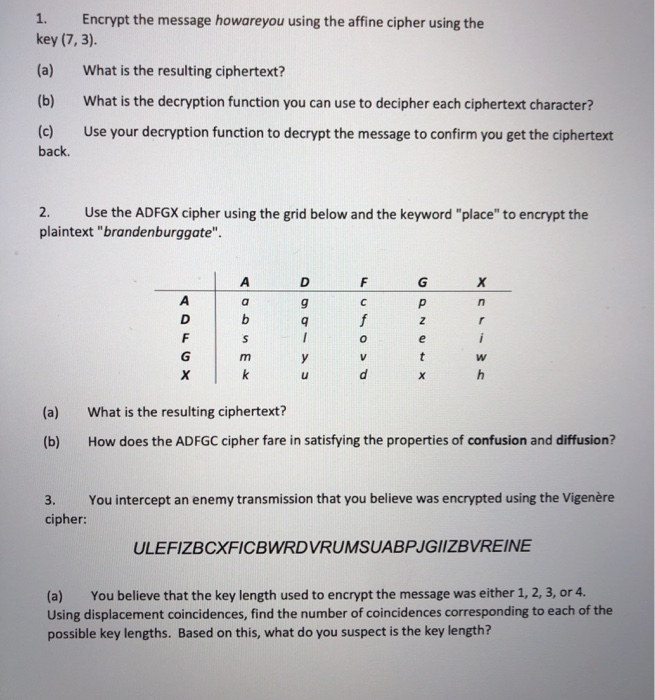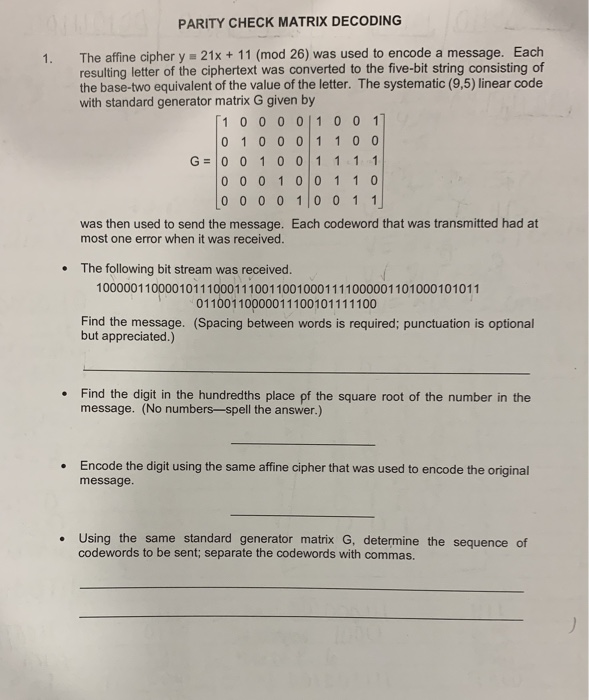Question

# shift and affine cipher program in Java ND c

shift and affine cipher program in Java ND c

Affine Cipher problem :

Affine cipher is a type of monoalphabetic substitution cipher,

wherein each letter in an alphabet is mapped to its numeric equivalent, encrypted using a simple mathematical function, and converted back to a letter. The formula used means that each letter encrypts to one other letter, and back again, meaning the cipher is essentially a standard substitution cipher with a rule governing which letter goes to which.

The whole process relies on working modulo m (the length of the alphabet used).

In the affine cipher, the letters of an alphabet of size m are first mapped to the integers in the range 0 … m-1.
The ‘key’ for the Affine cipher consists of 2 numbers, we’ll call them a and b. The following discussion assumes the use of a 26 character alphabet (m = 26).

A should be chosen to be relatively prime to m (i.e. a should have no factors in common with m).

Instructions while running code :

Please save file name as AffineCipher.java

Compile it using command javac AffineCipher.java

After successful compilation execute it using java AffineCipher

Java Source Code :

`import java.util.Scanner;`
`public class AffineCipher`
`{`
`    public static String encryptionMessage(String Msg,int a,int b)`
`    {`
`        String CTxt = "";        `
`        for (int i = 0; i < Msg.length(); i++)`
`        {`

// encryption going on here

`            CTxt = CTxt + (char) ((((a * Msg.charAt(i)) + b) % 26) + 65);`
`        }`
`        return CTxt;`
`    }`
` `
`    public static String decryptionMessage(String CTxt,int a,int b)`
`    {`
`        String Msg = "";        `
`        int a_inv = 0;`
`        int flag = 0;`
`        for (int i = 0; i < 26; i++)`
`        {`
`            flag = (a * i) % 26;`
`            if (flag == 1)`
`            {`
`                a_inv = i;`
`                System.out.println(i);`
`            }`
`        }`
`        for (int i = 0; i < CTxt.length(); i++)`
`        {`

// message decryption going on here

`            Msg = Msg + (char) (((a_inv * ((CTxt.charAt(i) - b)) % 26)) + 65);`
`        }`
`        return Msg;`
`    }`
` `
`    public static void main(String[] args)`
`    {`
`        Scanner sc = new Scanner(System.in);`

int a,b;

`        System.out.println("Enter the message: ");`
`        String message = sc.next();`
```        System.out.println("Enter values of a and b :");
a=sc.nextInt();
b=sc.nextInt();
System.out.println("Message is :" + message);```
`        System.out.println("Encrypted Message is : "`
`                + encryptionMessage(message,a,b));`
`        System.out.println("Decrypted Message is: "`
`                + decryptionMessage(encryptionMessage(message,a,b)));`
`        sc.close();`
`    }`
`}`

// end of java source code

C source code :

```#include<stdio.h>
#include<string.h>
#include<ctype.h>
#include<stdlib.h>

int CalcGCD(int);
void main()
{
int i,j,k,gcd,a,b,numstr,numcipher;
char str,cipher;
printf("Enter a string\n");
gets(str);
//converting entered string to Capital letters
for(i=0,j=0;i<strlen(str);i++)
{
if(str[i]!=' ')
{
str[j]=toupper(str[i]);
j++;
}
else
{
str[j]=' ';
j++;
}
}
str[j]='\0';
printf("Entered string is : %s \n",str);
printf("Enter a value and must be between 1 and 25 both included\n");
scanf("%d",&a);
//Checking consitions
if(a<1 || a>25)
{
printf("a should lie in between 1 and 25\nSorry Try again !\n");
exit(0);
}
gcd=CalcGCD(a);
if(gcd!=1)
{
printf("gcd(a,26)=1 but \n gcd(%d,26)=%d\nSorry Try again !\n",a,gcd);
exit(0);
}
printf("Enter b value and must be between 0 and 25 both included\n");
scanf("%d",&b);
if(b<0 || b>25)
{
printf("b value should lie between 0 and 25\nSorry Try again !\n");
exit(0);
}
//Conditions Over
//Program Starts
//Storing string in terms of ascii and to restore spaces I used -20
for(i=0;i<strlen(str);i++)
{
if(str[i]!=' ')
numstr[i]=str[i]-'A';
else
numstr[i]=-20;
}
//Ciphering Process
//If numcipher is more than 25 .We need to convert and ensure that lie in between 0 and 25.(indicating Alphabets)
//A-0,B-1,C-2,.....Y-24,Z-25
printf("Affine Cipher text is\n");
for(i=0;i<strlen(str);i++)
{
if(numstr[i]!=-20)
{
numcipher[i]=((alpha*numstr[i])+b)%26;
printf("%c",(numcipher[i]+'A'));
}
else
{
printf(" ");
}
}
printf("\n");

}

int CalcGCD(int a)
{
int x;
int temp1=a;
int temp2=26;

while(temp2!=0)
{
x=temp2;
temp2=temp1%temp2;
temp1=x;
}
return(temp1);
}```

// end of cpp source code

#### Earn Coins

Coins can be redeemed for fabulous gifts.

Similar Homework Help Questions
• ### In cryptography, a Caesar cipher, also known as Caesar's cipher, the shift cipher, Caesar's code or...

In cryptography, a Caesar cipher, also known as Caesar's cipher, the shift cipher, Caesar's code or Caesar shift, is one of the simplest and most widely known encryption techniques. It is a type of substitution cipher in which each letter in the plaintext is replaced by a letter some fixed number of positions down the alphabet. Given an arbitrary cipher text file, you need to write a C++ program to find out the value of the shift, and decrypt the...

• ### using the website repl.it (must be done in Javascript) PGM #1 Write a Java program that can perform the Caesar cipher fo...

using the website repl.it (must be done in Javascript) PGM #1 Write a Java program that can perform the Caesar cipher for English messages that include both upper and lowercase alphabetic characters. The Caesar cipher replaces each plaintext letter with a different one, by a fixed number of places down the alphabet. The cipher illustrated here uses a left shift of three, so that (for example) each occurrence of E in the plaintext becomes B in the ciphertext. For example...

• ### 6.12. For the affine cipher in Chapter 1 the multiplicative inverse of an element modulo 26...6.12. For the affine cipher in Chapter 1 the multiplicative inverse of an element modulo 26 can be found as aamod 26. Derive this relationship by using Euler's Theorem.

• ### Create a C program: A caesar cipher is a simple cipher that shifts letters in a...

Create a C program: A caesar cipher is a simple cipher that shifts letters in a string. For example, shifting “ab” over by 1 would result in “bc”, and shifting “xyz” over by 2 would result in “zab”. The caesar program should take, in the command line an integer k, the amount to shift some text by, and a string f i l e, the name of a file containing text to encode using the cipher. For example, suppose “secret.txt”...

• ### 1. Encrypt the message howareyou using the affine cipher using the key (7,3). (a) What is...1. Encrypt the message howareyou using the affine cipher using the key (7,3). (a) What is the resulting ciphertext? (b) What is the decryption function you can use to decipher each ciphertext character? (c) Use your decryption function to decrypt the message to confirm you get the ciphertext back. 2. Use the ADFGX cipher using the grid below and the keyword "place" to encrypt the plaintext "brandenburggate". FREE GX (a) (b) What is the resulting ciphertext? How does the ADFGC...

• ### PARITY CHECK MATRIX DECODING 1. The affine cipher y 21x + 11 (mod 26) was used...PARITY CHECK MATRIX DECODING 1. The affine cipher y 21x + 11 (mod 26) was used to encode a message. Each resulting letter of the ciphertext was converted to the five-bit string consisting of the base-two equivalent of the value of the letter. The systematic (9,5) linear code with standard generator matrix G given by [1 0 0 0 0 1 0 0 11 To 1000 1100l G= 0 0 1 0 0 1 1 1 1 0 0 0...

• ### Change the following Shift Cipher program so that it uses OOP(constructor, ect.) import java.util...

Change the following Shift Cipher program so that it uses OOP(constructor, ect.) import java.util.*; import java.lang.*; /** * * @author STEP */ public class ShiftCipher { /** * @param args the command line arguments */ public static void main(String[] args) { // TODO code application logic here Scanner input = new Scanner(System.in); String plainText; System.out.print("Please enter your string: "); // get message plainText = input.nextLine(); System.out.print("Please enter your shift cipher key: "); // get s int s = input.nextInt(); int...

• ### Python program Use the provided shift function to create a caesar cipher program. Your program s...

python program Use the provided shift function to create a caesar cipher program. Your program should have a menu to offer the following options: Read a file as current message Save current message Type in a new message Display current message "Encrypt" message Change the shift value For more details, see the comments in the provided code. NO GLOBAL VARIABLES! Complete the program found in assignment.py. You may not change any provided code. You may only complete the sections labeled:#YOUR...

• ### Show that if only a single character is encrypted, then the shift cipher is perfectly secret

Show that if only a single character is encrypted, then the shift cipher is perfectly secret

Please help me write this Java program. I had posted this question before, but got an answer that was totally wrong. We are using the latest version of Java8. Thank You! -------------------------------------------------------------------------------------- Write a Java program that can successfully DECRYPT a string inputted by the user which has been encrypted using a Caesar Cipher with a unknown shift value(key). You can use brute force to do a for loop through all the 26 shift values, however, your program should only...